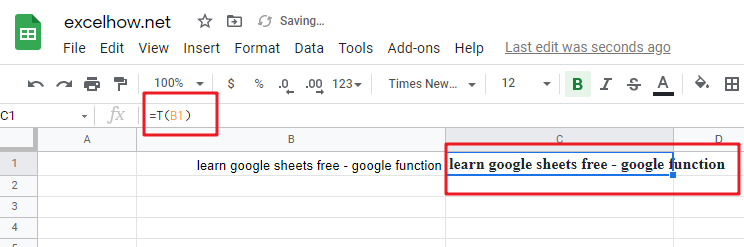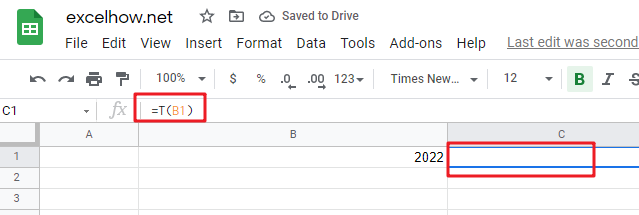This post will guide you how to use Google Sheets T function with syntax and examples.

## Description

The Google Sheets T function returns the text referred to by value. You can use T function to remove values that are not text.

The T function can be used to return text when given a text value as numbers, dates or the logic values in google sheets.  The purpose of this function is to filter text values only. And its returned output is also a text.

The T function is a build-in function in Google Sheets and it is categorized as a Text Function.

## Syntax

The syntax of the T function is as below:

= T (value)

Where the T function argument is:

• value -This is a required argument. The value you want to be converted text.

Note:

• The T function only takes one argument, `value`, which can be a cell reference, or a text value.
• If the `value` does not refer to a text string, the T function will return an empty text.
• If `value` is text, T function returns `value`.
• If `value` is a cell reference that containing text value, T function returns the content of `value`.
• If `value` is an error or a cell that containing an error, T function returns the error.

## Google Sheets T Function Examples

The below examples will show you how to use google sheets T function to return the text string referred to by value.

#1 To return the text string value in B1, just using formula:

`=T(B1) // it returns “learn google sheets free - google function”`#2 to return the text string value in cell B1 that containing a numreic value, using the following T function:

`=T(B1)  //it returns “”`Note: The T function is unnecessary. As Google sheets will convert between most formats automatically. And it is provided for compatibility with formulas used in other spreadsheet programs.